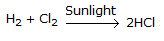# Chemical Engineering - Chemical Reaction Engineering

Exercise : Chemical Reaction Engineering - Section 6
1.
The order of the reaction,, is
0
1
2
3
Explanation:
No answer description is available. Let's discuss.

2.
Arhenious equation shows the variation of __________ with temperature.
reaction rate
rate constant
energy of activation
frequency factor
Explanation:
No answer description is available. Let's discuss.

3.
When a catalyst increases the rate of chemical reaction, the rate constant
decreases
increases
remains constant
becomes infinite
Explanation:
No answer description is available. Let's discuss.

4.
In which of the following reactions, the equilibrium will shift to the right, if the total pressure is increased?
H2 + Cl22HCl
N2 + O22NO
N2O42 NO2
H2 + I22HI